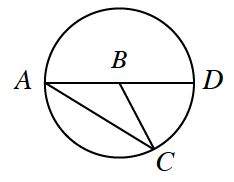### Home > INT2 > Chapter 10 > Lesson 10.2.1 > Problem10-53

10-53.

In the diagram below, $\overline{AD}$ is a diameter of $⊙B$. Each part is a new problem. Show all work.1. If $m∠A = 35°$, what is $m∠CBD$?

What is the relationship between the length of $AB$ and the length of $BC$?

Remember that a straight line has a total $m∠ of 180°$.

2. If $m∠CBD = 100°$, what is $m∠A$?

See hints for part (a).

$50°$

3. If $m∠A = x$, what is $m∠CBD$?

Notice the relationship between the given angles and their respective answers